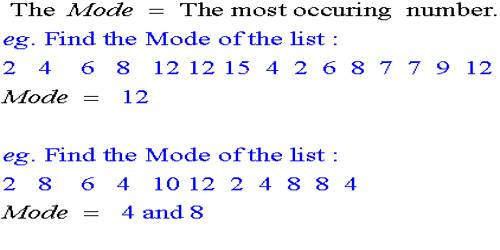Statistical Mode

The statistical mode is the number that occurs most frequently in a set of numbers. A statistical term that refers to the most frequently occurring number found in a set of numbers. The mode is found by collecting and organizing the data in order to count the frequency of each result.

It is a common measure of central tendency, along with median and means.

To find the mode of a group of numbers:

• Arrange the numbers in order by size.
• Determine the number of instances of each numerical value.
• The numerical value that has the most instances is the mode.
• There may be more than one mode when two or more numbers have an equal number of instances.
• A mode does not exist if no number has more than one instance.

Examples:

• The mode of the numbers 1, 2, 6, 6, 7 and 9 is 6
• There is no mode of the numbers 1, 2, 6, 7 and 9.
• The mode of the numbers 1, 2, 2, 6, 6, 7 and 9 is 6 and 2.The mode is the number that occurs most often within a set of numbers. For the server power consumption examples above, there is no mode because each element is different. But suppose the administrator measured the power consumption of an entire network operations center (NOC) and the set of numbers is 90 W, 104 W, 98 W, 98 W, 105 W, 92 W, 102 W, 100 W, 110 W, 98 W, 210 W and 115 W. The mode is 98 W since that power consumption measurement occurs most often amongst the 12 servers. Mode helps identify the most common or frequent occurrence of a characteristic. It is possible to have two modes (bimodal), three modes (trimodal) or more modes within larger sets of numbers.

In statistics, the mode in a list of numbers refers to the integers that occur most frequently.

Unlike the median and mean, the mode is about the frequency of occurrence. There can be more than one mode or no mode at all; it all depends on the dataset itself. For example, let’s say you have the following list of numbers:

3, 3, 8, 9, 15, 15, 15, 17, 17, 27, 40, 44, 44

In this case, the mode is 15 because it is the integer that appears most often. However, if there were one fewer 15 in your list, then you would have four modes: 3, 15, 17, and 44.

Explanation

For example, if the marks obtained by 15 students in a test are 81, 82, 85, 85, 89, 91, 91, 91, 91, 93, 93, 95, 96, 96 and 99, then the statistical mode for this data distribution is 91. This is because 4 students have obtained this score, which is the highest number of students with the same score.

It should be noted that unlike the mean and median, the mode doesn’t need to be unique. This is because there can always be two or more data points with the same frequency.

Consider a revised example for the test scores of the 15 students to be 81, 81, 85, 85, 89, 91, 91, 92, 92, 93, 93, 95, 96, 96 and 99. In this case, we see that 2 students score the same marks 81, 85, 91, 92 and 96, which are all the modes for this data distribution.

Information Source: